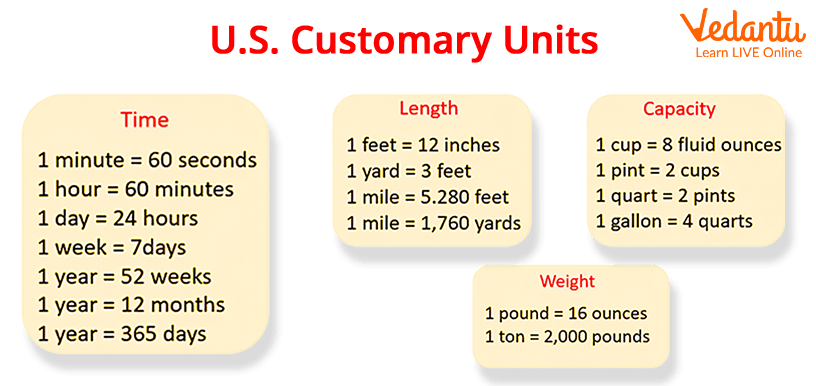Courses
Courses for Kids
Free study material
Free LIVE classes
More

# Customary Systems and Customary UnitsLIVE
Join Vedantu’s FREE Mastercalss

## What are Customary Systems?

Customary systems are the systems for weights and measures that are used in the United States and other countries. We find the usage of customary systems for measuring length, weight, capacity and temperature. These customary systems measure everything in terms of units. Like we measure length and distances in the customary system in inches, feet, yards and miles.

For example, a system based on the yard is a unit of length, the pound is the unit of weight, the gallon is the unit of liquid volume, and the bushel is the unit of dry volume.

Here, on this page, we will cover more about the customary system and the units in detail.Key Concepts of US-Based Customary Units

## Table for Customary Equivalent of Length and Distances

 Customary Unit Customary Equivalent Standard Metric Equivalent 1 inch - 2.54 cm 1 foot 12 inches 0.3048 meters 1 yard 3 feet 0.9144 meters 1 mile 1760 yards 1.609 344 kilometers

## Table for the US Customary Weight Units

 Customary Unit Customary Equivalent Standard Metric Equivalent 1 ounce 16 drams 28.349523125 gms 1 pound 16 ounces 453.59237 gms 1 ton 2000 pounds 907.18474 kgs

## Table for the U.S Customary Capacity or Volume Measurement Units

 Customary Unit Customary Equivalent Standard Metric Equivalent 1 fluid ounce 2 tablespoons 29.5735295625 milliliters 1 cup 8 fluid ounces 0236.5882365 milliliters 1 pint 2 cups 473.176473 milliliters 2 pins 0.946352946 liters 1 gallon 4 quarts 3.785411784 liters

## Customary Units for Temperature Measurement

The U.S. customary unit for temperature measurement is °Fahrenheit. So, the °F value is given as;

1 °C = 33.8 °F

## MCQs on Customary Systems and Units

1. 8 yards is equal to how many feet?

1. 23

2. 21

3. 24

4. 26

We know that 1 yard = 3 feet, so 8 feet = 3 times of yards value, i.e., 8 x 3 = 24.

Therefore, 8 yards is equal to 24 feet is the answer.

2. How many ounces make 12 pounds?

1. 80

2. 56

3. 64

4. 192

We know that 1 pound is equal to 16 ounces. Here, we have to find ‘?’ ounces to make 12 pounds. Let us solve this problem:

1 pound = 16 ounces

? = 12 pounds

16 12

16 (10 + 2)

(16 10) + (16 2)

160 + 32

=192

So, 192 ounces make 12 pounds, which is our required answer among the four options.

3. 2 gallons equal how many pints?

1. 16 pints

2. 18 pints

3. 19 pints

4. 20 pints

1 gallon is equal to 9.6076 U.S. liquid pint. So, 2 gallons = 12 times the 9.6076 U.S. liquid pint. So, we have

2 9.6076

=(2 9.0000) + (2 0.6076)

= 18.0000 + 1.2152

= 19.2152 approx. equal to 19 pints

So, our answer is option C i.e., 19 pints.

4. Convert 5°C into Fahrenheits.

1. 338 °F

2. 3.38 °F

3. 190 °F

4. 0.338 °F

We know that 1°C = 38 °F, so 5°C becomes 5 times the value of 38°F, so here we have:

5 38

= 5 (30 + 8)

=(5 30) + (5 8)

=150 + 40

= 190

So, the desired answer of the °F equivalent for 5°C is 190°F.

This was all about customary systems and their equivalent units for lengths and distances, volume, and temperature. Going through the MCQs solved above will help you give a clear understanding of this concept.

Last updated date: 28th Sep 2023
Total views: 127.8k
Views today: 1.27k

## FAQs on Customary Systems and Customary Units

1. How many units does the customary system have?

For measuring length and distances, the U.S. customary system holds the units of the inch, foot, yard, and mile, where these four are the length measurements in everyday use.

Since 1 July 1959, these customary units have been defined on the basis of 1 yard = 0.9144 meters excluding some surveying applications.

2. What do you conclude about the customary systems of measurement?

Customary systems state that when we go from a larger unit of measure to a smaller unit of measure we just need to multiply the given number by the conversion factor.